# Statistics Econometrics Statistics Econometrics Statistics Econometrics Statistics for

• Slides: 15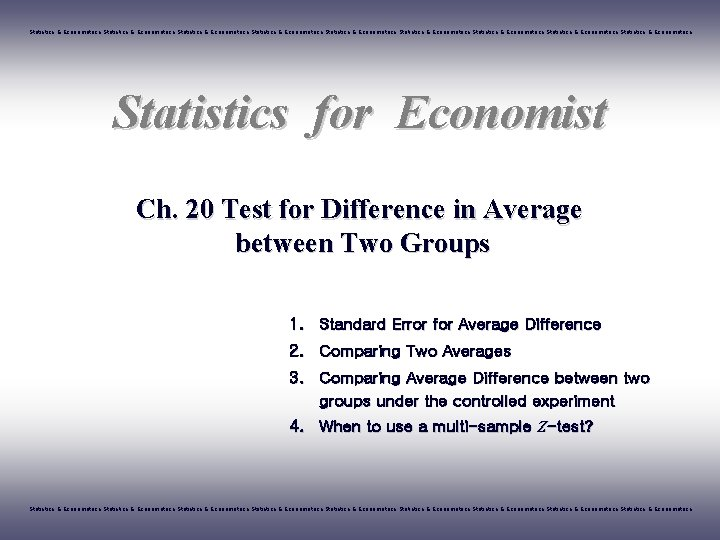Statistics & Econometrics Statistics & Econometrics Statistics & Econometrics Statistics for Economist Ch. 20 Test for Difference in Average between Two Groups 1. Standard Error for Average Difference 2. Comparing Two Averages 3. Comparing Average Difference between two groups under the controlled experiment 4. When to use a multi-sample Z-test? Statistics & Econometrics Statistics & Econometrics Statistics & EconometricsINDEX STATISTICS 1 Standard Error for Average Difference 2 Comparing Two Averages 3 Comparing Average Difference between two groups under the controlled experiment 4 When to use a multi-sample Z-test? Statistics & Econometrics Statistics & Econometrics Statistics & Econometrics 21. Standard Error for Average Difference STATISTICS S. E. for Difference in Groups Ø Random Replacement Sampling Box A Aver. 120 S. D. 60 Box B Aver. 80 S. D. 40 Aver. of Box A from 400 times drawing out = 120 3 Aver. of Box A from 100 times drawing out = 80 4 - If Two Groups were Independent, S. E. of Average difference is - a, b are S. E. of each average Statistics & Econometrics Statistics & Econometrics Statistics & Econometrics 3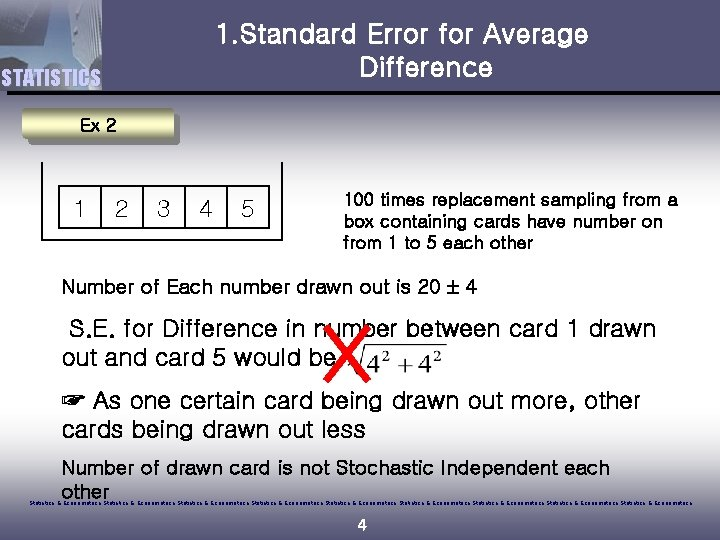1. Standard Error for Average Difference STATISTICS Ex 2 1 2 3 4 5 100 times replacement sampling from a box containing cards have number on from 1 to 5 each other Number of Each number drawn out is 20 4 S. E. for Difference in number between card 1 drawn out and card 5 would be ☞ As one certain card being drawn out more, other cards being drawn out less Number of drawn card is not Stochastic Independent each other Statistics & Econometrics Statistics & Econometrics Statistics & Econometrics 4INDEX STATISTICS 1 Standard Error for Average Difference 2 Comparing Two Averages 3 Comparing Average Difference between two groups under the controlled experiment 4 When to use a multi-sample Z-test? Statistics & Econometrics Statistics & Econometrics Statistics & Econometrics 5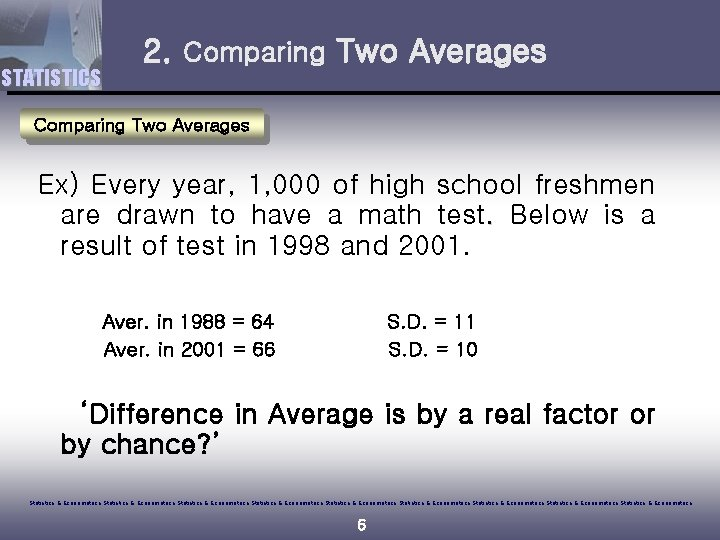STATISTICS 2. Comparing Two Averages Ex) Every year, 1, 000 of high school freshmen are drawn to have a math test. Below is a result of test in 1998 and 2001. Aver. in 1988 = 64 Aver. in 2001 = 66 S. D. = 11 S. D. = 10 ‘Difference in Average is by a real factor or by chance? ’ Statistics & Econometrics Statistics & Econometrics Statistics & Econometrics 6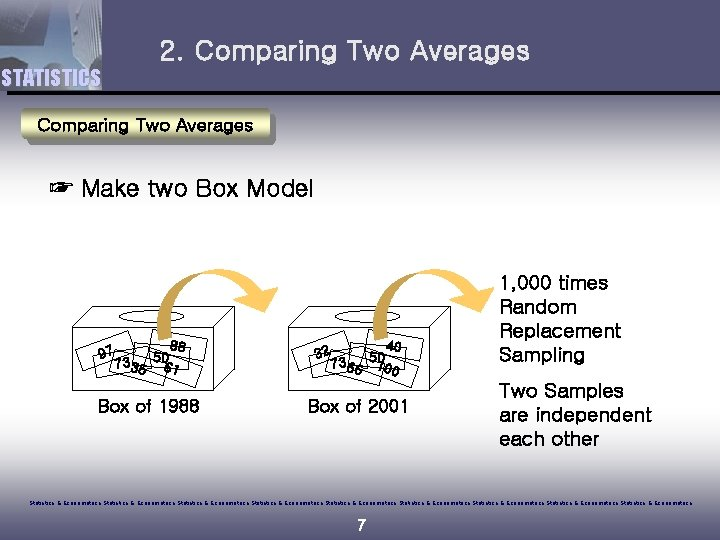2. Comparing Two Averages STATISTICS Comparing Two Averages ☞ Make two Box Model 97 88 5 0 7335 61 Box of 1988 32 40 5 0 7366 100 Box of 2001 1, 000 times Random Replacement Sampling Two Samples are independent each other Statistics & Econometrics Statistics & Econometrics Statistics & Econometrics 7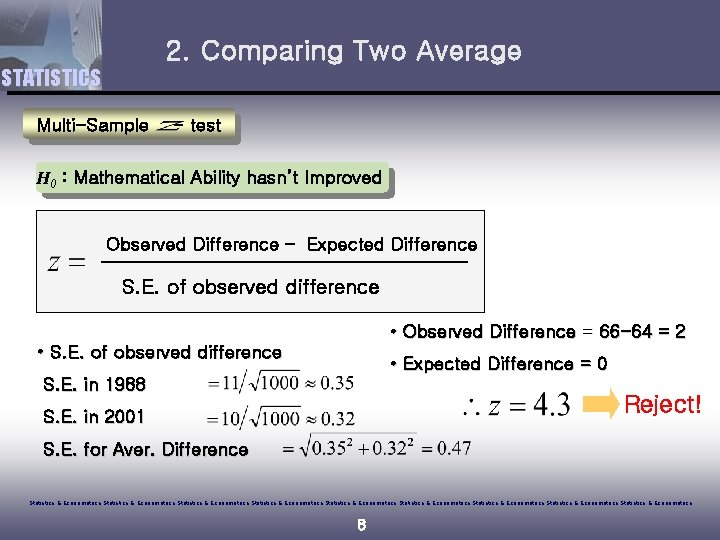2. Comparing Two Average STATISTICS Multi-Sample - test H 0 : Mathematical Ability hasn’t Improved Observed Difference – Expected Difference S. E. of observed difference • Observed Difference = 66 -64 = 2 • S. E. of observed difference • Expected Difference = 0 S. E. in 1988 Reject! S. E. in 2001 S. E. for Aver. Difference Statistics & Econometrics Statistics & Econometrics Statistics & Econometrics 8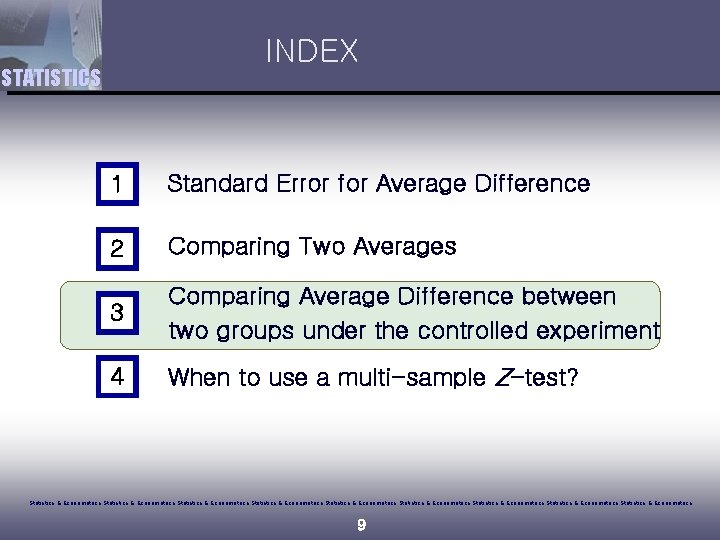INDEX STATISTICS 1 Standard Error for Average Difference 2 Comparing Two Averages 3 Comparing Average Difference between two groups under the controlled experiment 4 When to use a multi-sample Z-test? Statistics & Econometrics Statistics & Econometrics Statistics & Econometrics 9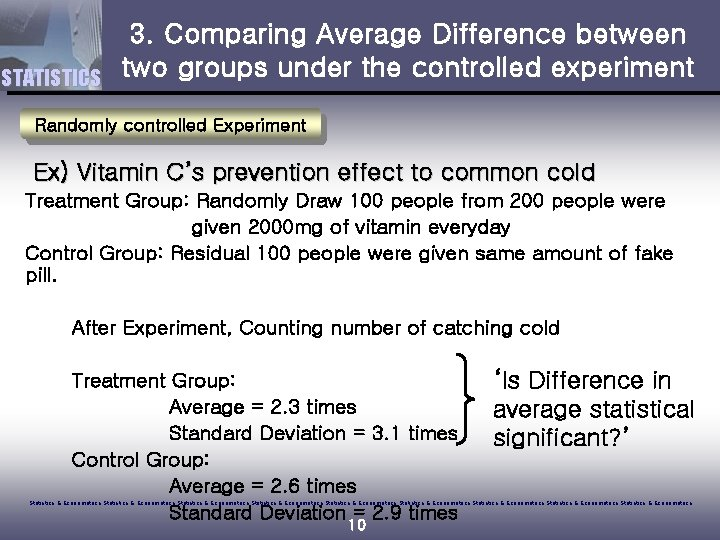STATISTICS 3. Comparing Average Difference between two groups under the controlled experiment Randomly controlled Experiment Ex) Vitamin C’s prevention effect to common cold Treatment Group: Randomly Draw 100 people from 200 people were given 2000 mg of vitamin everyday Control Group: Residual 100 people were given same amount of fake pill. After Experiment, Counting number of catching cold Treatment Group: Average = 2. 3 times Standard Deviation = 3. 1 times Control Group: Average = 2. 6 times Standard Deviation = 2. 9 times ‘Is Difference in average statistical significant? ’ Statistics & Econometrics Statistics & Econometrics Statistics & Econometrics 10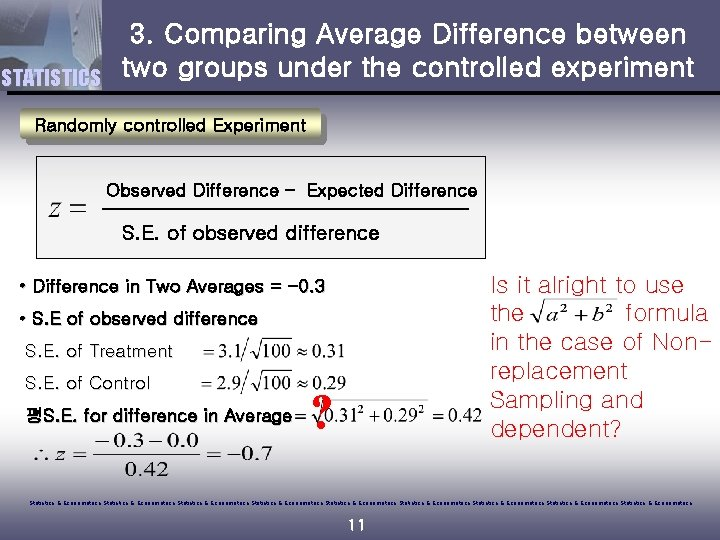STATISTICS 3. Comparing Average Difference between two groups under the controlled experiment Randomly controlled Experiment Observed Difference – Expected Difference S. E. of observed difference Is it alright to use the formula in the case of Nonreplacement Sampling and dependent? • Difference in Two Averages = -0. 3 • S. E of observed difference S. E. of Treatment S. E. of Control 평S. E. for difference in Average ? Statistics & Econometrics Statistics & Econometrics Statistics & Econometrics 11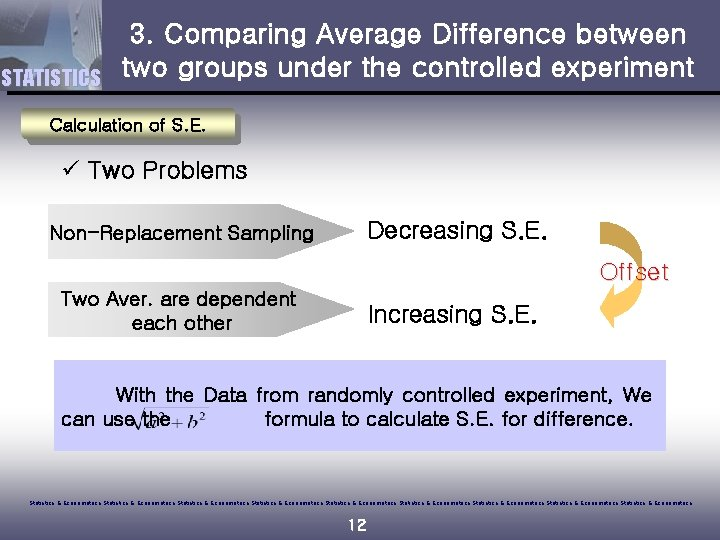STATISTICS 3. Comparing Average Difference between two groups under the controlled experiment Calculation of S. E. ü Two Problems Decreasing S. E. Non-Replacement Sampling Offset Two Aver. are dependent each other Increasing S. E. With the Data from randomly controlled experiment, We can use the 무작위로 formula to calculate S. E. for difference. Statistics & Econometrics Statistics & Econometrics Statistics & Econometrics 12INDEX STATISTICS 1 Standard Error for Average Difference 2 Comparing Two Averages 3 Comparing Average Difference between two groups under the controlled experiment 4 When to use a multi-sample Z-test? Statistics & Econometrics Statistics & Econometrics Statistics & Econometrics 13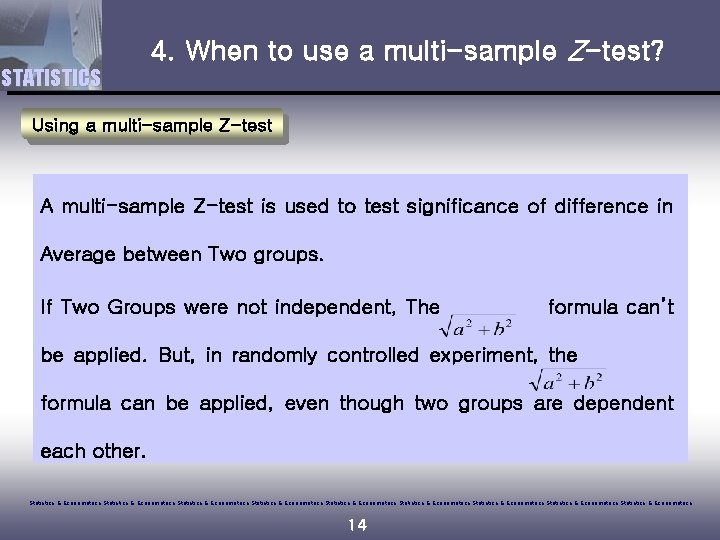4. When to use a multi-sample Z-test? STATISTICS Using a multi-sample Z-test A multi-sample Z-test is used to test significance of difference in Average between Two groups. If Two Groups were not independent, The 두 표본이formula can’t be applied. But, in randomly controlled experiment, the 무작위로 formula can be applied, even though two groups are dependent each other. Statistics & Econometrics Statistics & Econometrics Statistics & Econometrics 14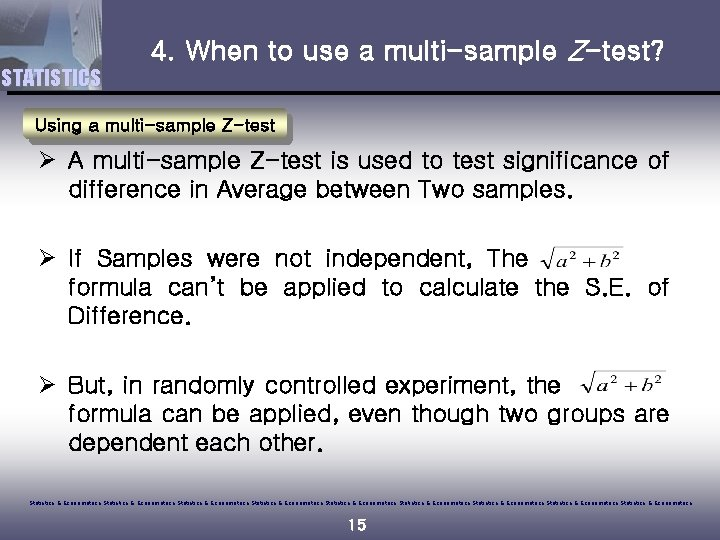4. When to use a multi-sample Z-test? STATISTICS Using a multi-sample Z-test Ø A multi-sample Z-test is used to test significance of difference in Average between Two samples. Ø If Samples were not independent, The 두 표 본 이 formula can’t be applied to calculate the S. E. of Difference. Ø But, in randomly controlled experiment, the 무작위로 formula can be applied, even though two groups are dependent each other. Statistics & Econometrics Statistics & Econometrics Statistics & Econometrics 15